# Problem 4For the shown structure, AB is made by A-36 steel (Est = 200GPA, ast = 12 x 10-67 °C) and BC is made by%|73.1GPA, aal = 23 × 10-6/ °C). Both AB and BC are having circular cross sectional area. Thealuminum (Ealgap between C and the rigid wall at D is initially 0.15 mm. For the following situations, find (1) reaction forces atfixed end A and D (2) Plot the axial load diagramP=400 kN applied at B and there is no temperature-0.15 тm600 mm600 mmvi.changevii.P=400 kN applied and temperature in both AB and BC25°Csegments had dropped ATP=400 kN applied, however, the temperature in ABincreased ATst = 25°C and temperature in BCdecreased ATal = 25°Cvii.B.25 mm50 mm

Question
8 views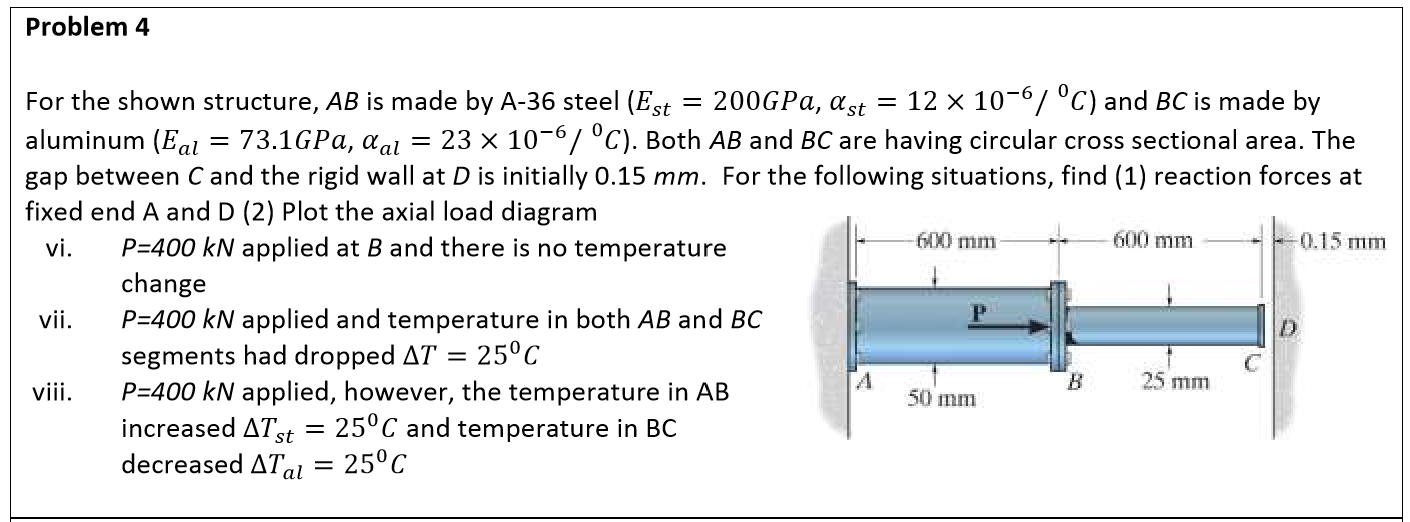help_outlineImage TranscriptioncloseProblem 4 For the shown structure, AB is made by A-36 steel (Est = 200GPA, ast = 12 x 10-67 °C) and BC is made by %| 73.1GPA, aal = 23 × 10-6/ °C). Both AB and BC are having circular cross sectional area. The aluminum (Eal gap between C and the rigid wall at D is initially 0.15 mm. For the following situations, find (1) reaction forces at fixed end A and D (2) Plot the axial load diagram P=400 kN applied at B and there is no temperature -0.15 тm 600 mm 600 mm vi. change vii. P=400 kN applied and temperature in both AB and BC 25°C segments had dropped AT P=400 kN applied, however, the temperature in AB increased ATst = 25°C and temperature in BC decreased ATal = 25°C vii. B. 25 mm 50 mm fullscreen
check_circle

Step 1

The free-body diagram of the structure is shown below.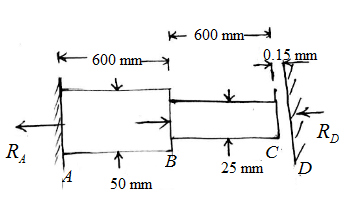Consider the equilibrium of forces in the horizontal direction.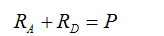Step 2

vi)

Given P = 400 kN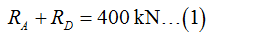The elongation in a rod is given by,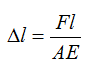Here, F is the force, l is the length of the rod, A is the area of the rod and E is the modulus of elasticity.

Step 3

The total elongation of the structure is 0.15 mm as there is no temperature change.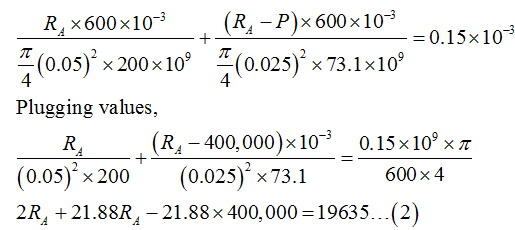...

### Want to see the full answer?

See Solution

#### Want to see this answer and more?

Solutions are written by subject experts who are available 24/7. Questions are typically answered within 1 hour.*

See Solution
*Response times may vary by subject and question.
Tagged in